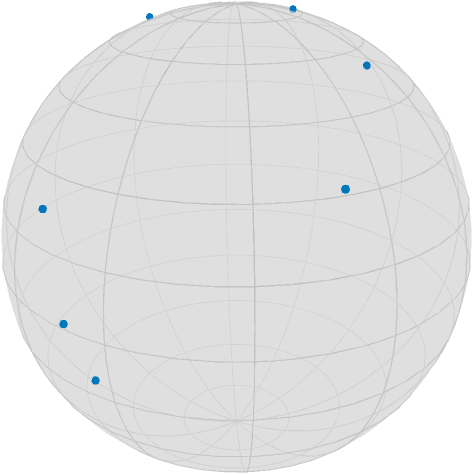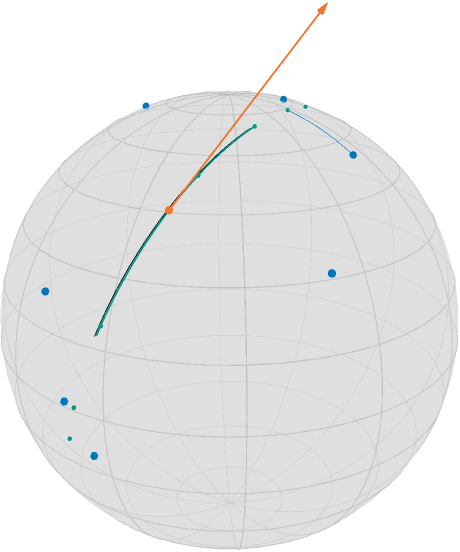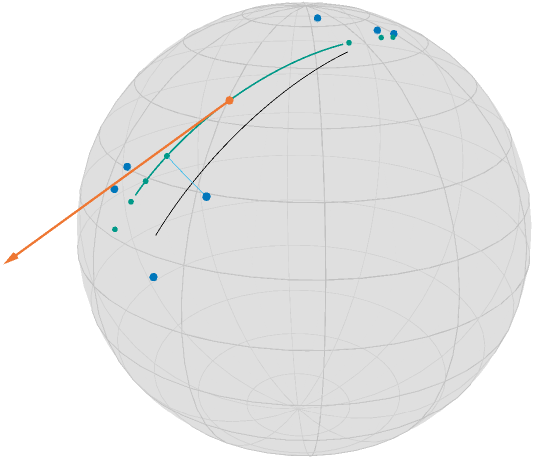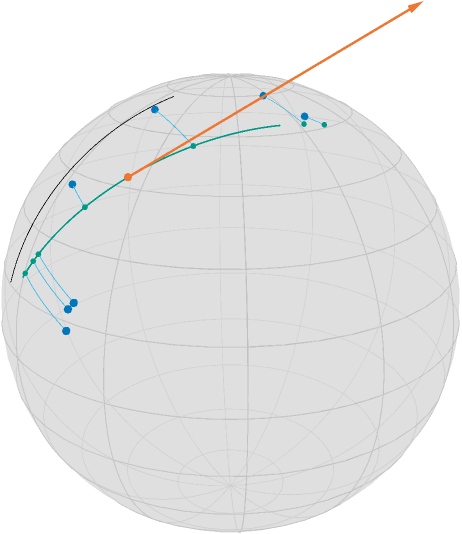# Geodesic Regression

Geodesic regression generalizes linear regression to Riemannian manifolds. Let's first phrase it informally as follows:

For given data points $d_1,\ldots,d_n$ on a Riemannian manifold $\mathcal M$ find the geodesic that “best explains” the data.

The meaning of “best explain” has still to be clarified. We distinguish two cases: time labelled data and unlabelled data

using Manopt, Manifolds, Colors, Random, PlutoUI
using LinearAlgebra: svd

We define some colors from Paul Tol.

begin
black = RGBA{Float64}(colorant"#000000")
TolVibrantOrange = RGBA{Float64}(colorant"#EE7733")
TolVibrantBlue = RGBA{Float64}(colorant"#0077BB")
TolVibrantTeal = RGBA{Float64}(colorant"#009988")
TolVibrantMagenta = RGBA{Float64}(colorant"#EE3377")
TolVibrantCyan = RGBA{Float64}(colorant"#33BBEE")
Random.seed!(42)
end;

We use the following data, where you can choose one data point, that you wakt to highlight.

begin
n = 7
highlighted = 4
(highlighted > n - 1) && error(
"Please choose a highlighted point from {1,...,$(n-1)} – you set it to highlighted.", ) σ = π / 8 S = Sphere(2) base = 1 / sqrt(2) * [1.0, 0.0, 1.0] dir = [-0.75, 0.5, 0.75] data_orig = [exp(S, base, dir, t) for t in range(-0.5, 0.5; length=n)] # add noise to the points on the geodesic data = map(x -> exp(S, x, random_tangent(S, x, :Gaussian, σ)), data_orig) localpath = join(splitpath(@__FILE__)[1:(end - 1)], "/") # files folder image_prefix = localpath * "/regression" @info image_prefix render_asy = false # on CI or when you do not have asymptote, this should be false end; This notebook loads data if render_asymptote is set to false, and renders the images (using Asymptote) otherwise. render_asy && asymptote_export_S2_signals( image_prefix * "/regression_data.asy"; points=[data], colors=Dict(:points => [TolVibrantBlue]), dot_size=3.5, camera_position=(1.0, 0.5, 0.5), ); render_asy && render_asymptote(image_prefix * "/regression_data.asy"; render=2); PlutoUI.LocalResource(image_prefix * "/regression_data.png")## Time labeled data if for each data item$d_i$we are also given a time point$t_i\in\mathbb R$, which are pairwise different. Then we can use the least squares error to state the objetive function as [Fletcher2013] $$F(p,X) = \frac{1}{2}\sum_{i=1}^n d_{\mathcal M}^2(γ_{p,X}(t_i), d_i),$$ where$d_{\mathcal M}$is the Riemannian distance and$γ_{p,X}$is the geodesic with$γ(0) = p$and$\dot\gamma(0) = X$. For the real-valued case$\mathcal M = \mathbb R^m$the solution$(p^*, X^*)$is given in closed form as follows: with$d^* = \frac{1}{n}\displaystyle\sum_{i=1}^{n}d_i$and$t^* = \frac{1}{n}\displaystyle\sum_{i=1}^n t_i$we get $$X^* = \frac{\sum_{i=1}^n (d_i-d^*)(t-t^*)}{\sum_{i=1}^n (t_i-t^*)^2} \quad\text{ and }\quad p^* = d^* - t^*X^*$$ and hence the linear regression result is the line$γ_{p^*,x^*}(t) = p^* + tX^*$. On a Riemannian manifold we can phrase this as an optimization problem on the tangent bundle, i.e. the disjoiint union of all tangent spaces, as $$\operatorname*{arg\,min}_{(p,X) \in \mathrm{T}\mathcal M} F(p,X)$$ Due to linearity, the gradient of$F(p,X)$is the sum of the single gradients of $$\frac{1}{2}d_{\mathcal M}^2\bigl(γ_{p,X}(t_i),d_i\bigr) = \frac{1}{2}d_{\mathcal M}^2\bigl(\exp_p(t_iX),d_i\bigr) ,\quad i∈\{1,\ldots,n\}$$ which can be computed using a chain rule of the squared distance and the exponential map, see for example [BergmannGousenbourger2018] for details or Equations (7) and (8) of [Fletcher2013]: M = TangentBundle(S) TangentBundle(Sphere(2, ℝ)) begin struct RegressionCost{T,S} data::T times::S end RegressionCost(data::T, times::S) where {T,S} = RegressionCost{T,S}(data, times) function (a::RegressionCost)(M, x) pts = [geodesic(M.manifold, x[M, :point], x[M, :vector], ti) for ti in a.times] return 1 / 2 * sum(distance.(Ref(M.manifold), pts, a.data) .^ 2) end end; begin struct RegressionGradient!{T,S} data::T times::S end function RegressionGradient!(data::T, times::S) where {T,S} return RegressionGradient!{T,S}(data, times) end function (a::RegressionGradient!)(M, Y, x) pts = [geodesic(M.manifold, x[M, :point], x[M, :vector], ti) for ti in a.times] gradients = grad_distance.(Ref(M.manifold), a.data, pts) Y[M, :point] .= sum( adjoint_differential_exp_basepoint.( Ref(M.manifold), Ref(x[M, :point]), [ti * x[M, :vector] for ti in a.times], gradients, ), ) Y[M, :vector] .= sum( adjoint_differential_exp_argument.( Ref(M.manifold), Ref(x[M, :point]), [ti * x[M, :vector] for ti in a.times], gradients, ), ) return Y end end; Now we need just a start point. For the Euclidean case, the result is given by the first principal component of a principal component analysis, see PCR, i.e. with$p^* = \frac{1}{n}\displaystyle\sum_{i=1}^n d_i$the direction$X^*$is obtained by defining the zero mean data matrix $$D = \bigl(d_1-p^*, \ldots, d_n-p^*\bigr) \in \mathbb R^{m,n}$$ and taking$X^*$as an eigenvector to the larges eigenvalue of$D^{\mathrm{T}}D$. We can do something similar, when considering the tangent space at the (Riemannian) mean of the data and then do a PCA on the coordinate coefficients with respect to a basis. begin m = mean(S, data) A = hcat( map(x -> get_coordinates(S, m, log(S, m, x), DefaultOrthonormalBasis()), data)... ) pca1 = get_vector(S, m, svd(A).U[:, 1], DefaultOrthonormalBasis()) x0 = ProductRepr(m, pca1) end ProductRepr with 2 submanifold components: Component 1 = 3-element Vector{Float64}: 0.7484132473986155 -0.0034768578817765544 0.663223584153586 Component 2 = 3-element Vector{Float64}: 0.4707886138814662 -0.7015690308438468 -0.5349382917686802 The optimal “time labels” are then just the projections$t_i = ⟨d_i,X^*⟩$,$i=1,\ldots,n$. t = map(d -> inner(S, m, pca1, log(S, m, d)), data) 7-element Vector{Float64}: 0.6064193661761539 0.5527730243506181 -0.4623704108489327 -0.18820463944210314 -0.10368999657043663 0.06575987939344873 -0.484834440664573 And we can call the gradient descent. Note that since gradF! works in place of Y, we have to set the evalutation type accordingly. with_terminal() do global y = gradient_descent( M, RegressionCost(data, t), RegressionGradient!(data, t), x0; evaluation=MutatingEvaluation(), stepsize=ArmijoLinesearch(1.0, ExponentialRetraction(), 0.99, 0.1), stopping_criterion=StopAfterIteration(100) | StopWhenGradientNormLess(1e-8), debug=[:Iteration, " | ", :Cost, "\n", :Stop, 50], ) end Initial | F(x): 0.158782 # 50 | F(x): 0.158545 # 100 | F(x): 0.158545 The algorithm reached its maximal number of iterations (100).  The result looks like begin dense_t = range(-0.5, 0.5; length=100) geo = geodesic(S, y[M, :point], y[M, :vector], dense_t) init_geo = geodesic(S, x0[M, :point], x0[M, :vector], dense_t) geo_pts = geodesic(S, y[M, :point], y[M, :vector], t) geo_conn_highlighted = shortest_geodesic( S, data[highlighted], geo_pts[highlighted], 0.5 .+ dense_t ) end 100-element Vector{Vector{Float64}}: [0.4094849811312494, -0.15158608140556787, 0.8996353206450072] [0.41233112922123094, -0.14874468936540716, 0.8988092440894971] [0.4151703268638402, -0.14590079001482992, 0.897968016782792] [0.4180025262002268, -0.14305443129194073, 0.897111652905015] [0.4208276794895074, -0.14020566117630043, 0.8962401668914383] [0.42364573910956954, -0.13735452768811743, 0.8953535734322395] [0.42645665755787565, -0.13450107888743826, 0.8944518874722551] ⋮ [0.6406294526311229, 0.11995679897478362, 0.7584222246224533] [0.642631213890406, 0.12282127650926236, 0.756267186231413] [0.6446221426558135, 0.12568368371055963, 0.7540993998451212] [0.6466022053673144, 0.12854397232859388, 0.7519189020048046] [0.6485713686480405, 0.13140209414899542, 0.7497257294659602] [0.65052959930485, 0.1342580009939191, 0.7475199191977349] render_asy && asymptote_export_S2_signals( image_prefix * "/regression_result1.asy"; points=[data, [y[M, :point]], geo_pts], curves=[init_geo, geo, geo_conn_highlighted], tangent_vectors=[[Tuple([y[M, :point], y[M, :vector]])]], colors=Dict( :curves => [black, TolVibrantTeal, TolVibrantBlue], :points => [TolVibrantBlue, TolVibrantOrange, TolVibrantTeal], :tvectors => [TolVibrantOrange], ), dot_sizes=[3.5, 3.5, 2], line_widths=[0.33, 0.66, 0.33, 1.0], camera_position=(1.0, 0.5, 0.5), ); render_asy && render_asymptote(image_prefix * "/regression_result1.asy"; render=2); PlutoUI.LocalResource(image_prefix * "/regression_result1.png")In this image, together with the blue data points, you see the geodesic of the initialization in black (evaluated on$[-\frac{1}{2},\frac{1}{2}]$), the final point on the tangent bundle in orange, as well as the resulting regression geodesic in teal, (on the same interval as the start) as well as small teal points indicating the time points on the geodesic corresponding to the data. Additionally, a thin blue line indicates the geodesic between a data point and its corresponding data point on the geodesic. While this would be the closest point in Euclidean space and hence the two directions (along the geodesic vs. to the data point) orthogonal, here we have inner( S, geo_pts[highlighted], log(S, geo_pts[highlighted], geo_pts[highlighted + 1]), log(S, geo_pts[highlighted], data[highlighted]), ) -0.0008204813969674813 But we also started with one of the best scenarios, i.e. equally spaced points on a geodesic obstructed by noise this gets worse if you start with less even distributed data begin data2 = [exp(S, base, dir, t) for t in [-0.5, -0.49, -0.48, 0.1, 0.48, 0.49, 0.5]] data2 = map(x -> exp(S, x, random_tangent(S, x, :Gaussian, σ / 2)), data2) m2 = mean(S, data2) A2 = hcat( map(x -> get_coordinates(S, m, log(S, m, x), DefaultOrthonormalBasis()), data2)... ) pca2 = get_vector(S, m, svd(A2).U[:, 1], DefaultOrthonormalBasis()) x1 = ProductRepr(m, pca2) t2 = map(d -> inner(S, m2, pca2, log(S, m2, d)), data2) end 7-element Vector{Float64}: 0.6374507553785159 0.9011915071556038 0.39236962150910004 -0.21263752851357945 -0.4502492314619214 -0.629174920007797 -0.6612984074116082 with_terminal() do global y2 = gradient_descent( M, RegressionCost(data2, t2), RegressionGradient!(data2, t2), x1; evaluation=MutatingEvaluation(), stepsize=ArmijoLinesearch(1.0, ExponentialRetraction(), 0.990, 0.05), stopping_criterion=StopAfterIteration(200) | StopWhenGradientNormLess(1e-8), debug=[:Iteration, " | ", :Cost, "\n", :Stop, 50], ) end Initial | F(x): 0.053741 # 50 | F(x): 0.052822 # 100 | F(x): 0.052822 # 150 | F(x): 0.052822 # 200 | F(x): 0.052822 The algorithm reached its maximal number of iterations (200).  begin geo2 = geodesic(S, y2[M, :point], y2[M, :vector], dense_t) init_geo2 = geodesic(S, x1[M, :point], x1[M, :vector], dense_t) geo_pts2 = geodesic(S, y2[M, :point], y2[M, :vector], t2) geo_conn_highlighted2 = shortest_geodesic( S, data2[highlighted], geo_pts2[highlighted], 0.5 .+ dense_t ) end 100-element Vector{Vector{Float64}}: [0.6333320446214238, 0.24007436599422644, 0.735700224308867] [0.6331834177808643, 0.23853735129341486, 0.7363278423944497] [0.633033031698058, 0.23699967383837753, 0.7369534146604304] [0.6328808867908391, 0.23546133790141066, 0.7375769393687136] [0.6327269834819279, 0.23392234775664003, 0.738198414786893] [0.6325713221989311, 0.23238270768000915, 0.7388178391882559] [0.6324139033743391, 0.23084242194926716, 0.7394352108517883] ⋮ [0.6117423085003225, 0.09327568461653696, 0.785538665280082] [0.6114307899544987, 0.09169525399933195, 0.7859671554776418] [0.6111175726041161, 0.09011456861524555, 0.7863934619373059] [0.6108026573194208, 0.08853363285606777, 0.7868175834746205] [0.6104860449753756, 0.08695245111428393, 0.7872395189112027] [0.6101677364516594, 0.08537102778306302, 0.7876592670747434] render_asy && asymptote_export_S2_signals( image_prefix * "/regression_result2.asy"; points=[data2, [y2[M, :point]], geo_pts2], curves=[init_geo2, geo2, geo_conn_highlighted2], tangent_vectors=[[Tuple([y2[M, :point], y2[M, :vector]])]], colors=Dict( :curves => [black, TolVibrantTeal, TolVibrantCyan], :points => [TolVibrantBlue, TolVibrantOrange, TolVibrantTeal], :tvectors => [TolVibrantOrange], ), dot_sizes=[3.5, 3.5, 2.5], line_widths=[0.33, 0.66, 0.33, 1.0], camera_position=(1.0, 0.5, 0.5), ); render_asy && render_asymptote(image_prefix * "/regression_result2.asy"; render=2); PlutoUI.LocalResource(image_prefix * "/regression_result2.png")## Unlabeled data If we are not given time points$t_i$, then the optimization problem extends – informally speaking – to also finding the “best fitting” (in the sense of smallest error). To formalize, the objective function here reads $$F(p, X, t) = \frac{1}{2}\sum_{i=1}^n d_{\mathcal M}^2(γ_{p,X}(t_i), d_i),$$ where$t = (t_1,\ldots,t_n) \in \mathbb R^n$is now an additional parameter of the objective function. We write$F_1(p, X)$to refer to the function on the tangent bundle for fixed values of$t$(as the one in the last part) and$F_2(t)$for the function$F(p, X, t)$as a function in$t$with fixed values$(p, X)$. For the Euclidean case, there is no neccessity to optimize with respect to$t$, as we saw above for the initialisation of the fixed time points. On a Riemannian manifold this can be stated as a problem on the product manifold$\mathcal N = \mathrm{T}\mathcal M \times \mathbb R^n$, i.e. N = M × Euclidean(length(t2)) ProductManifold with 2 submanifolds: TangentBundle(Sphere(2, ℝ)) Euclidean(7; field = ℝ) $$\operatorname*{arg\,min}_{\bigl((p,X),t\bigr)\in\mathcal N} F(p, X, t).$$ In this tutorial we present an approach to solve this using an alternating gradient descent scheme. To be precise, we define the cost funcion now on the product manifold begin struct RegressionCost2{T} data::T end RegressionCost2(data::T) where {T} = RegressionCost2{T}(data) function (a::RegressionCost2)(N, x) TM = N pts = [ geodesic(TM.manifold, x[N, 1][TM, :point], x[N, 1][TM, :vector], ti) for ti in x[N, 2] ] return 1 / 2 * sum(distance.(Ref(TM.manifold), pts, a.data) .^ 2) end end The gradient in two parts, namely (a) the same gradient as before w.r.t.$(p,X) ∈ T\mathcal M$just now with a fixed t in mind for the second component of the product manifold$\mathcal N$begin struct RegressionGradient2a!{T} data::T end RegressionGradient2a!(data::T) where {T} = RegressionGradient2a!{T}(data) function (a::RegressionGradient2a!)(N, Y, x) TM = N p = x[N, 1] pts = [geodesic(TM.manifold, p[TM, :point], p[TM, :vector], ti) for ti in x[N, 2]] gradients = grad_distance.(Ref(TM.manifold), a.data, pts) Y[TM, :point] .= sum( adjoint_differential_exp_basepoint.( Ref(TM.manifold), Ref(p[TM, :point]), [ti * p[TM, :vector] for ti in x[N, 2]], gradients, ), ) Y[TM, :vector] .= sum( adjoint_differential_exp_argument.( Ref(TM.manifold), Ref(p[TM, :point]), [ti * p[TM, :vector] for ti in x[N, 2]], gradients, ), ) return Y end end Finally we addionally look for a fixed point$x=(p,X) ∈ \mathrm{T}\mathcal M$at the gradient with respect to$t∈\mathbb R^n\$, i.e. the second component, which is given by

$$(\operatorname{grad}F_2(t))_i = - ⟨\dot γ_{p,X}(t_i), \log_{γ_{p,X}(t_i)}d_i⟩_{γ_{p,X}(t_i)}, i = 1, \ldots, n.$$

begin
data::T
end
TM = N
p = x[N, 1]
pts = [geodesic(TM.manifold, p[TM, :point], p[TM, :vector], ti) for ti in x[N, 2]]
logs = log.(Ref(TM.manifold), pts, a.data)
pt = map(
d -> vector_transport_to(TM.manifold, p[TM, :point], p[TM, :vector], d), pts
)
Y .= -inner.(Ref(TM.manifold), pts, logs, pt)
return Y
end
end

We can reuse the computed initial values from before, just that now we are on a product manifold

begin
x2 = ProductRepr(x1, t2)
F3 = RegressionCost2(data2)
end;
with_terminal() do
N,
F3,
x2;
evaluation=MutatingEvaluation(),
debug=[:Iteration, " | ", :Cost, "\n", :Stop, 50],
stepsize=ArmijoLinesearch(1.0, ExponentialRetraction(), 0.999, 0.066, 1e-11),
inner_iterations=1,
)
end
Initial  | F(x): 0.053741
# 50     | F(x): 0.052555
# 100    | F(x): 0.052555
The algorithm reached its maximal number of iterations (100).

begin
geo3 = geodesic(S, y3[N, 1][M, :point], y3[N, 1][M, :vector], dense_t)
init_geo3 = geodesic(S, x1[M, :point], x1[M, :vector], dense_t)
geo_pts3 = geodesic(S, y3[N, 1][M, :point], y3[N, 1][M, :vector], y3[N, 2])
t3 = y3[N, 2]
geo_conns = shortest_geodesic.(Ref(S), data2, geo_pts3, Ref(0.5 .+ dense_t))
end;
render_asy && asymptote_export_S2_signals(
image_prefix * "/regression_result3.asy";
points=[data2, [y3[N, 1][M, :point]], geo_pts3],
curves=[init_geo3, geo3, geo_conns...],
tangent_vectors=[[Tuple([y3[N, 1][M, :point], y3[N, 1][M, :vector]])]],
colors=Dict(
:curves =>
[black, TolVibrantTeal, repeat([TolVibrantCyan], length(geo_conns))...],
:points => [TolVibrantBlue, TolVibrantOrange, TolVibrantTeal],
:tvectors => [TolVibrantOrange],
),
dot_sizes=[3.5, 3.5, 2.5],
line_widths=[0.33, 0.66, repeat([0.33], length(geo_conns))..., 1.0],
camera_position=(1.0, 0.5, 0.5),
);
render_asy && render_asymptote(image_prefix * "/regression_result3.asy"; render=2);
PlutoUI.LocalResource(image_prefix * "/regression_result3.png")Note that the geodesics from the data to the regression geodesic meet at an nearly orthogonal angle.

Acknowledgement. Parts of this tutorial are based on the bachelor thesis of Jeremias Arf.

## References

BergmannGousenbourger2018

Bergmann, R. and Gousenbourger, P.-Y.: A variational model for data fitting on manifolds by minimizing the acceleration of a Bézier curve. Frontiers in Applied Mathematics and Statistics, 2018. doi: 10.3389/fams.2018.00059, arXiv: 1807.10090

Fletcher2013

Fletcher, P. T., Geodesic regression and the theory of least squares on Riemannian manifolds, International Journal of Computer Vision(105), 2, pp. 171–185, 2013. doi: 10.1007/s11263-012-0591-y